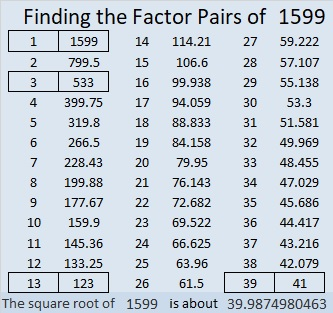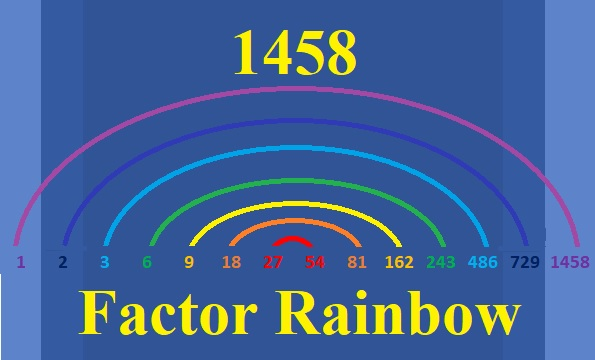# 1599 and Level 5

### Today’s Puzzle:

Write the numbers 1 to 10 in both the first column and the top row so that this level 5 puzzle will function like a multiplication table. Use logic with every step.### Factors of 1599:

• 1599 is a composite number.
• Prime factorization: 1599 = 3 × 13 × 41.
• 1599 has no exponents greater than 1 in its prime factorization, so √1599 cannot be simplified.
• The exponents in the prime factorization are 1, 1, and 1. Adding one to each exponent and multiplying we get (1 + 1)(1 + 1)(1 + 1) = 2 × 2 × 2 = 8. Therefore 1599 has exactly 8 factors.
• The factors of 1599 are outlined with their factor pair partners in the graphic below.### More about the Number 1599:

1599 is the hypotenuse of FOUR Pythagorean triples:
276-1575-1599, which is 3 times (92-525-533),
351-1560-1599, which is 39 times (9-40-41),
615-1476-1599, which is (5-12-13) times 123, and
924-1305-1599, which is 3 times (308-435-533).

1599 is the difference of two squares four different ways:
800² – 799² = 1599,
268² – 265² = 1599,
68² – 55² = 1599, and
40² – 1² = 1599.
Yes, we are just one number away from a perfect square!

# 1458 Tangrams Can Be A Pot of Gold

### A Tangram Puzzle

Tangrams are seven puzzle pieces that can form a square but can also be made into many different people, places, and things. A lot of stress is going on in the world right now, but since tomorrow is Saint Patrick’s Day, we can still find a little pot of gold at the end of the rainbow!

I made this pot of gold on Desmos using points and equations. If you cut it apart, will you be able to put it back together again?What other things can you make from those seven tangram shapes?

And what about that rainbow I mentioned? The number 1458 makes a lovely factor rainbow.

#### A Factor Rainbow for 1458:#### Factors of the number 1458:

• 1458 is a composite number.
• Prime factorization: 1458 = 2 × 3 × 3 × 3 × 3 × 3 × 3, which can be written 1458 = 2 × 3⁶.
• 1458 has at least one exponent greater than 1 in its prime factorization so √1458 can be simplified. Taking the factor pair from the factor pair table below with the largest square number factor, we get √1458 = (√729)(√2) = 27√2
• The exponents in the prime factorization are 1 and 6. Adding one to each exponent and multiplying we get (1 + 1)(6 + 1) = 2 × 7 = 14. Therefore 1458 has exactly 14 factors.
• The factors of 1458 are outlined with their factor pair partners in the graphic below.#### More Facts about the Number 1458:

2 is a prime factor of 1458 exactly one time, so there are NO ways that 1458 can be written as the difference of two squares.

2 and 3 are the only primes appearing in its prime factorization, so 1458 is NEVER the hypotenuse of a Pythagorean triple.

Nevertheless, since there are three different ways that 1458 = 2(a)(b), where a > b, there are three ways that 1458 is a leg in a Pythagorean triple:
1458-531440-531442, calculated from 2(729)(1), 729² – 1², 729² + 1²
1458-59040-59058, calculated from 2(243)(3), 243² – 3², 243² + 3²
1458-6480-6642, calculated from 2(81)(9), 81² – 9², 81² + 9²

Why can’t we get a Pythagorean triple from 1458 = 2(27)(27)? I’m sure you can figure out that one yourself.

# 911 Mystery Level Puzzle

911 is a prime number that is also the sum of three consecutive primes:

• 293 + 307 + 311 = 911

911 is 191 in BASE 26 because 1(26²) + 9(26) + 1(1) = 911

911 is palindrome 12121 in BASE 5 because 1(5⁴) + 2(5³) + 1(5²) + 2(5) + 1(1) = 911Print the puzzles or type the solution on this excel file: 12 factors 905-913

• 911 is a prime number.
• Prime factorization: 911 is prime.
• The exponent of prime number 911 is 1. Adding 1 to that exponent we get (1 + 1) = 2. Therefore 911 has exactly 2 factors.
• Factors of 911: 1, 911
• Factor pairs: 911 = 1 × 911
• 911 has no square factors that allow its square root to be simplified. √911 ≈ 30.1827765

How do we know that 911 is a prime number? If 911 were not a prime number, then it would be divisible by at least one prime number less than or equal to √911 ≈ 30.2. Since 911 cannot be divided evenly by 2, 3, 5, 7, 11, 13, 17, 19, 23, or 29, we know that 911 is a prime number.